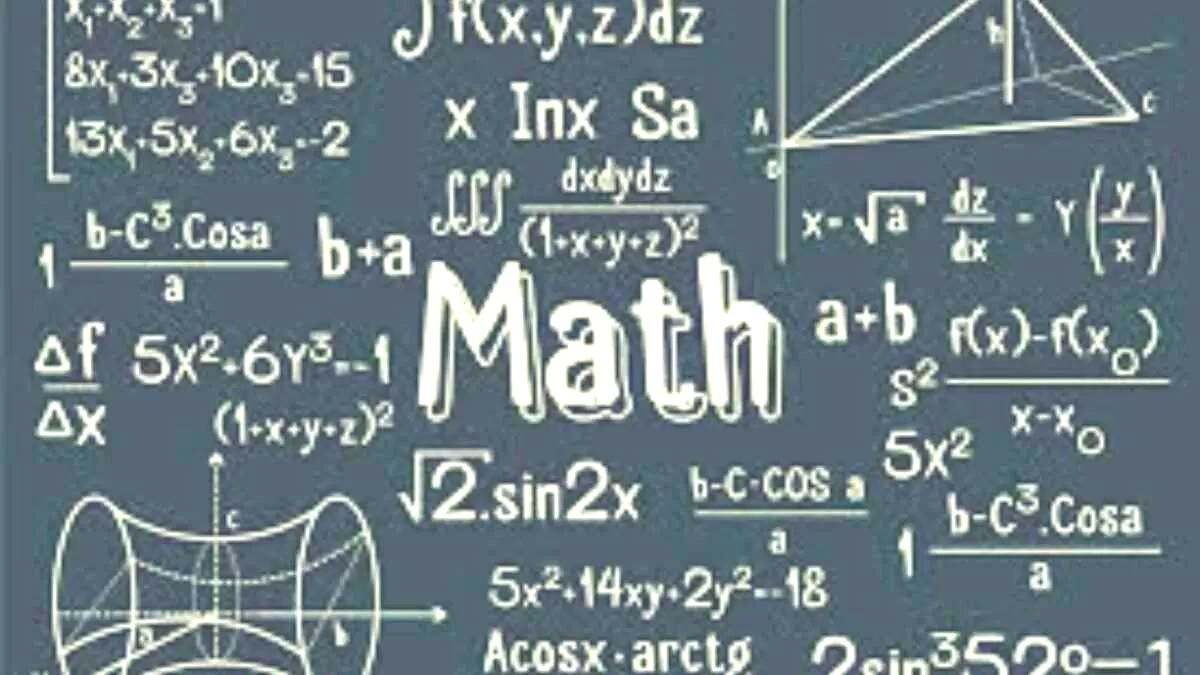# Math riddles with answers: These math riddles are fit for your tiny tots!

Math riddles are a fun and productive way to keep your children engaged. Make your tiny tots enjoy these fun math riddles.Have you ever tried stuffing green leafy vegetables in interesting burgers or other food items so your child eats them? That’s what you have to do with subjects like math.

Yes, math is a subject that is hated by most students but can be made enjoyable in the form of math riddles.

That is when you should try these math riddles with your kids!

MATH RIDDLES:

Math Riddle 1:

Jessica’s pencil was wearing out and is now only three centimeters long. Her friend Ross’s new pencil was eight times that length. How long was Ross’s pencil?

MATH RIDDLE 2

Imagine you are 8 feet away from a gate.  Additionally, with each step you take, you advance half the distance to the door. How many steps do you think will it take for you to reach the gate?

Math Riddle 3:

Do you know how to count from 1 to 1000? If yes, then which one-digit number appears most often between and including the numbers, 1 and 1,000? Put on your thinking caps, buddy!

Math Riddle 1:

Jessica’s pencil was wearing out and is now only three centimeters long. Her friend Ross’s new pencil was eight times that length. How long was Ross’s pencil?

8 multiplied by 3 is 24. So, the answer is 24 cm.

MATH RIDDLE 2

Imagine you are 8 feet away from a gate.  Additionally, with each step you take, you advance half the distance to the door. How many steps do you think will take for you to reach the gate?

Answer 2: The answer is Infinity! Think about it, can you ever reach the gate, since you can only step forward half the distance, irrespective of the size of the step?

Math Riddle 3:

Do you know how to count from 1 to 1000? If yes, then which one-digit number appears most often between and including the numbers, 1 and 1,000? Put on your thinking caps, buddy!